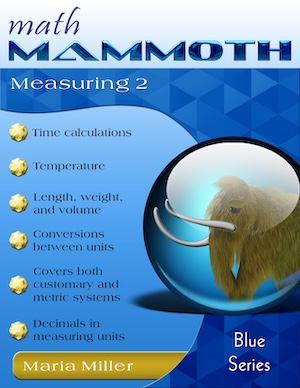^

# Free measuring videos online

Find here free online math videos on measurement-related topics, meant for about 4th grade level: measuring length, weight, and volume, customary and metric units, temperature, and time units. I also have a separate page with measurement video lessons for grades 1-3, which are, obviously, simpler.

You can use these videos...

• ...to learn these topics yourself (if you're a student for example, or an adult needing a refresher)
• ...as lesson plans for teaching these topics. Often, one video from below can be made into several lessons with students.

The videos match some of the lessons in my book Math Mammoth Measuring 2 (Blue Series book), or the lessons in chapter 4 of Grade 4-A (Light Blue series). In any of those books, you will get MANY more practice exercises, word problems, and puzzles than what are shown in the videos, and also some lessons that are not in covered in this set of videos.69 pages (includes answers) PDF download \$6.80 Printed copy \$11.60, b&w Math Mammoth Measuring 2 worktext Read more!

## Measuring length to the nearest 1/8 inch and in centimeters/millimeters

This is a lesson for about fourth grade level, where students are already familiar with centimeters and inches from the previous grades. I explain how to measure length with a ruler that has tick marks every 1/8 inch, and also with a ruler that measures in centimeters and millimeters. I also go through some conversion problems between centimeters and millimeters.

## Conversions between inches, feet, and yards

I explain how to change (convert) between an amount in feet an inches, and inches, such as 4 ft 6 in to inches. Then, we do it the other way around: an amount in inches into feet & inches, such as 56 in. to feet and inches.

We do the same with yards & feet to feet, and feet to yards & feet. Then I solve some perimeter word problems. Lastly I touch a little bit on miles versus feet.

## Metric units of length (from millimeter to kilometer)

I explain the metric units for length from millimeter to kilometer on a chart. Then I solve some conversion problems, and lastly a few word problems.

## Measuring weight in customary units

In the customary system, we have the units ounce, pound, and (short ton). I give some "ballpark" weights of various things, and then go on to solve simple conversion problems between the units and a few word problems. This lesson is intended for about fourth grade.

## Measuring Weight in the Metric System

We take a look at grams and kilograms for measuring weight in the metric system. I give some "ballpark" weights, and then we do some easy conversion problems. Lastly, I solve three different word problems -- the last one provided by Mathy, my mammoth mascot.

## Metric units of volume

The main units we use in the metric system for measuring volume are milliliter and liter. But actually there are two units between those: centiliter and deciliter. Then I solve some simple conversion problems between units, and several word problems.

## Measuring temperature - Celsius and Fahrenheit degrees

I compare Celsius and Fahrenheit scales for measuring temperature and give some ballpark temperatures for both. Lastly, I solve simple problems about rising or dropping temperatures using integers.

## Time units

A basic lesson about different time units (minutes, hours, days, months, years) for about 4th grade level. I solve a few conversion problems and several word problems involving time units.

The videos match some of the lessons in my book Math Mammoth Measuring 2 (Blue Series book), or the lessons in chapter 4 of Grade 4-A (Light Blue series). In any of those books, you will get MANY more practice exercises, word problems, and puzzles than what are shown in the videos, and also some lessons that are not in covered in this set of videos.69 pages (includes answers) PDF download \$6.80 Printed copy \$11.60, b&w Math Mammoth Measuring 2 worktext Read more!

By Maria Miller

WAIT!

Receive my monthly collection of math tips & resources directly in your inbox — and get a FREE Math Mammoth book!You can unsubscribe at any time.

### Math Mammoth Tour

Confused about the different options? Take a virtual email tour around Math Mammoth! You'll receive:

An initial email to download your GIFT of over 400 free worksheets and sample pages from my books. Six other "TOURSTOP" emails that explain the important things and commonly asked questions concerning Math Mammoth curriculum. (Find out the differences between all these different-colored series!)

This way, you'll have time to digest the information over one or two weeks, plus an opportunity to ask me personally about the curriculum.
A monthly collection of math teaching tips & Math Mammoth updates (unsubscribe any time)### "Mini" Math Teaching Course

This is a little "virtual" 2-week course, where you will receive emails on important topics on teaching math, including:

- How to help a student who is behind
- Troubles with word problems
- Teaching multiplication tables
- Why fractions are so difficult
- The value of mistakes
- Should you use timed tests
- And more!

A GIFT of over 400 free worksheets and sample pages from my books right in the very beginning.A monthly collection of math teaching tips & Math Mammoth updates (unsubscribe any time)
Enter your email to receive math teaching tips, resources, Math Mammoth news & sales, humor, and more! I tend to send out these tips about once monthly, near the beginning of the month, but occasionally you may hear from me twice per month (and sometimes less often).• A GIFT of over 400 free worksheets and sample pages from my books.